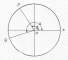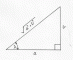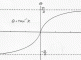I have forgotten

•http://facebook.com/
•https://www.google.com/accounts/o8/id
•https://me.yahoo.com

# Trigonometry

#### Formulae

General trigonometric identities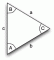#### Trigonometrical Ratios

Describes the derivation of the basic ratios and their relationship one with another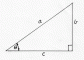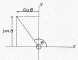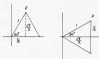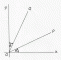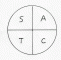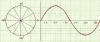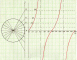#### The Trigonometry of the Triangle

The sine and cos formula; areas of triangles and an analysis of the varios circles associated with Triangles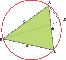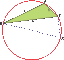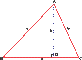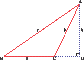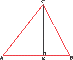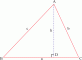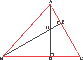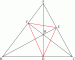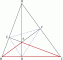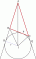#### Trigonometrical Formulae

A collection of formulae covering, Addition and subtraction of Sin cos and tan; Product formulae ; the solution of equations and the half angle formulae and the Inverser Ratioi